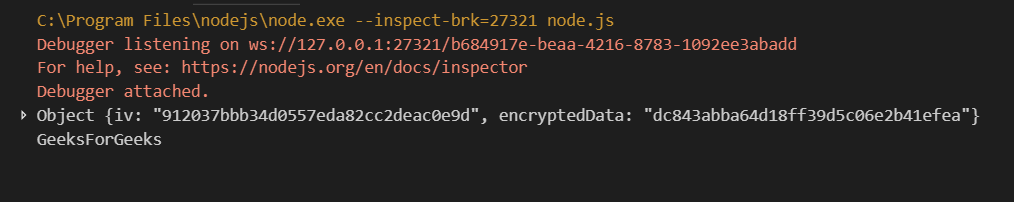Open in App
Not now

# Encrypting Data in Node.js

• Last Updated : 14 Oct, 2021

Encryption and Decryption in Node can be done by installing and implementing the ‘crypto’ library. If you have installed Node.js by manual build, then there is a chance that the crypto library is not shipped with it. You can run
the following command to install the crypto dependency.

`npm install crypto --save`

But you don’t need to do that if you have installed it using pre-built packages.
Example for implementing crypto in Node.

## javascript

 `// Nodejs encryption with CTR ` `const crypto = require(``'crypto'``); ` `const algorithm = ``'aes-256-cbc'``; ` `const key = crypto.randomBytes(32); ` `const iv = crypto.randomBytes(16); ` ` `  `function` `encrypt(text) { ` `let cipher = crypto.createCipheriv(``'aes-256-cbc'``,Buffer.from(key), iv); ` `let encrypted = cipher.update(text); ` `encrypted = Buffer.concat([encrypted, cipher.final()]); ` `return` `{ iv: iv.toString(``'hex'``), encryptedData: encrypted.toString(``'hex'``) }; ` `} ` ` `  `function` `decrypt(text) { ` `let iv = buffer.from(text.iv, ``'hex'``); ` `let encryptedText = Buffer.from(text.encryptedData, ``'hex'``); ` `let decipher = crypto.createDecipheriv(``'aes-256-cbc'``, Buffer.from(key), iv); ` `let decrypted = decipher.update(encryptedText); ` `decrypted = Buffer.concat([decrypted, decipher.final()]); ` `return` `decrypted.toString(); ` `} ` ` `  `var` `gfg = encrypt(``"GeeksForGeeks"``); ` `console.log(gfg); ` `console.log(decrypt(gfg)); `

OutputMy Personal Notes arrow_drop_up
Related Articles